Ram Dewani — September 27, 2020

## Overview

• Microsoft Excel is an excellent tool for learning and executing statistical functions
• Here are 12 statistical functions in Excel that you should master for a successful analytics career

## Let’s Excel in Statistics!

“Statistics is the grammar of Science.” – Karl  Pearson

Let’s make that a bit more relevant for us – Statistics is the grammar of “Data” Science. You’ll notice that almost every successful data science professional or analytics professional has a solid understanding of statistics – but does that mean you need a master’s degree in the subject?

Absolutely not!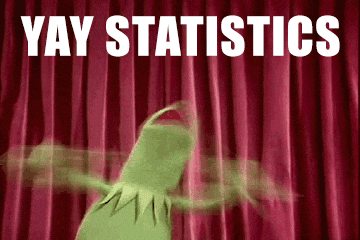We’ll approach this article using the famous Pareto Principle – 80% of the consequences come from 20% of the causes. Therefore, we’ll focus majorly on 20% of the concepts and functions that come in handy 80% of the time! Now, the question as an analyst is, how to get started with implementing statistics?

Sure, you can implement and practice these concepts in programming languages like Python and R. But hold your horses for a second and think – what’s the most commonly used tool in most organizations?

It’s Microsoft Excel! Honestly, Excel is the Swiss Army Knife for analytics professionals that helps you focus on what’s important (statistics in our case) and handles the rest of the calculations and customizations itself.

This article is for everyone who’s starting their journey into statistics using Excel. We will use 10 key statistical functions in Excel to answer questions for a dummy sports company, Khelo, while perusing at their data.

If you are a beginner in the world of analytics and Excel, I’d highly recommend going through these free courses:

## How We’ll Approach These 1o Statistical Functions in Excel

I have divided these statistical functions in Excel into two categories:

• Basic statistical functions
• Intermediate statistical functions

Let’s begin!

## Understanding the Data and the Problem Statement

We will be solving a few key questions about our dummy sports equipment and apparel company – Khelo. These are the columns we have:

• Equipment
• Number of items sold
• Cost of each item
• Discount %
• Revenue1. How many items are at a discount?
2. How many items/pieces of equipment are sold by the store?
3. What is the number of products sold without a discount?
4. Are there any products sold having cost more than 2000 along with a discount rate greater than 50%?
5. What is the average number of products sold?
6. What is the median of the number of products sold?
7. What is the most frequent discount percentage?
8. What is the standard deviation of the number of products sold?
9. Is there any relationship between the number of products sold and the discount percentage?

## Basic Statistical Functions in Excel

MS Excel provides an array of useful statistical functions. Let us begin with some of the basic yet extremely powerful functions. Honestly, you’ll find that you’re using the basic statistical functions 90% of the time and the rest 10% of your time is taken by intermediate and advanced functions.

We will be talking majorly about the different kinds of count functions here. These are very similar to other functions such as sum, max, min, average.

### 1. Count Function

We use the count function when we need to count the number of cells containing a number. Remember ONLY NUMBERS! Let’s see the function:

• COUNT(value1, [value2], …)

So, let’s try to find the answer to our first question – How many items were on discount?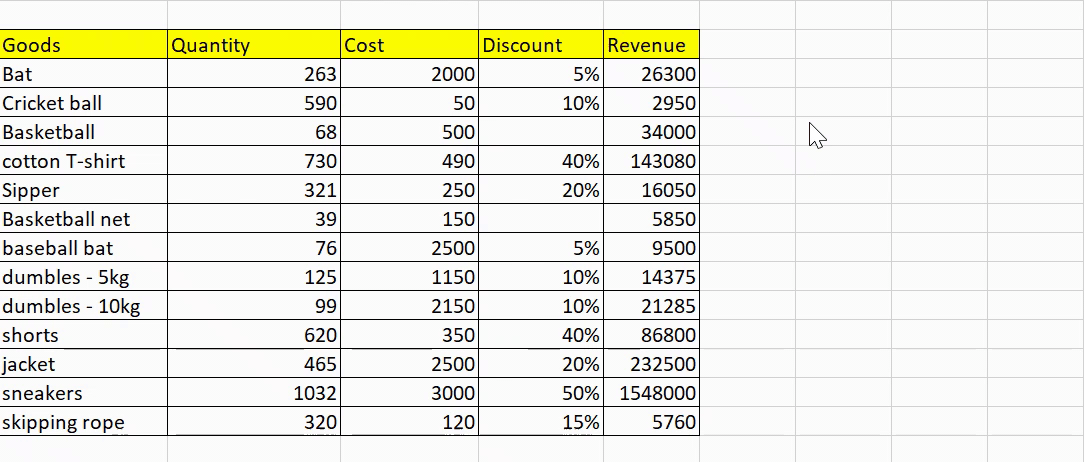There are 11 products on discount.

### 2. Counta Function

While the count function only counts the numeric values, the COUNTA function counts all the cells in a range that are not empty. The function is useful for counting cells containing any type of information, including error values and empty text.

• COUNTA(value1, [value2], …)
We’ll answer the second question using the counta function since it is able to count all the non-empty values – How many items/pieces of equipment are sold by the store?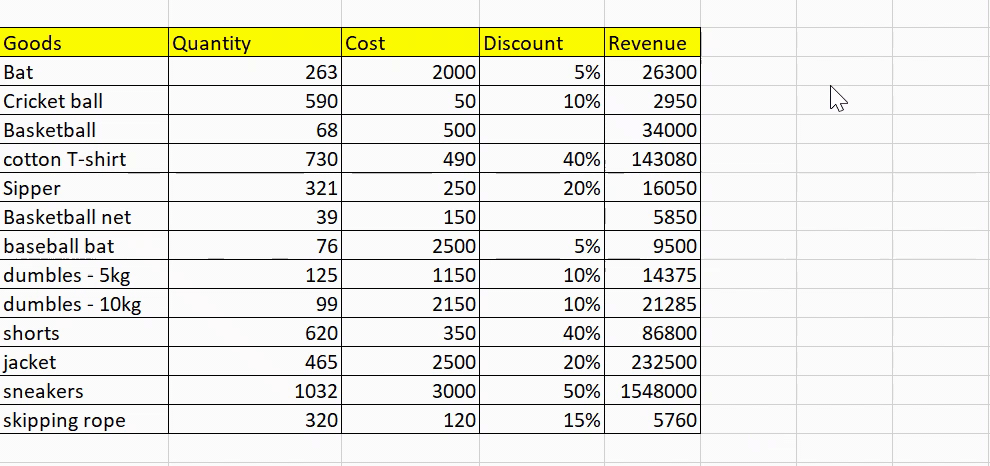The total number of items sold by the store is 13.

### 3. Countblank

The COUNTBLANK function counts the number of empty cells in a range of cells. Cells with formulas that return empty text are also counted here but cells with zero values are not counted. This is a great function for summarizing empty cells while analyzing any data.

• COUNTBLANK(range)
Summarizing empty cells is the requirement for our third question – What products are not in the discount section? Let’s apply the function!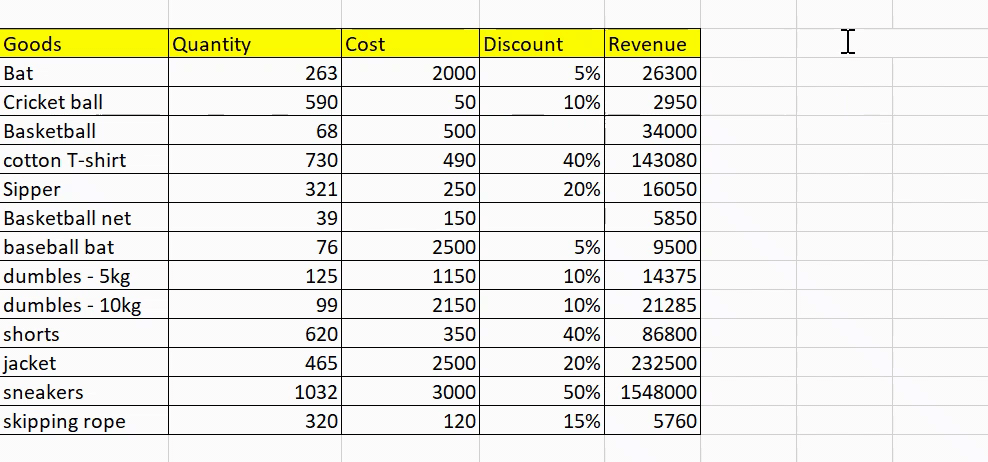There are only 2 items not on discount.

### 4. Countifs Function

Countifs are one of the most used statistical functions in Excel. The COUNTIFS function applies one or more conditions to the cells in the given range and returns only those cells that fulfill all of the conditions.

• COUNTIFS(criteria_range1, criteria1, [criteria_range2, criteria2]…)
Note: Every new range must have the same number of rows and columns as the criteria_range1 argument. The ranges do not have to be adjacent to each other.
This function seems perfect to answer the fourth question – Are there any products sold having cost more than 2000 along with a discount rate greater than 50%?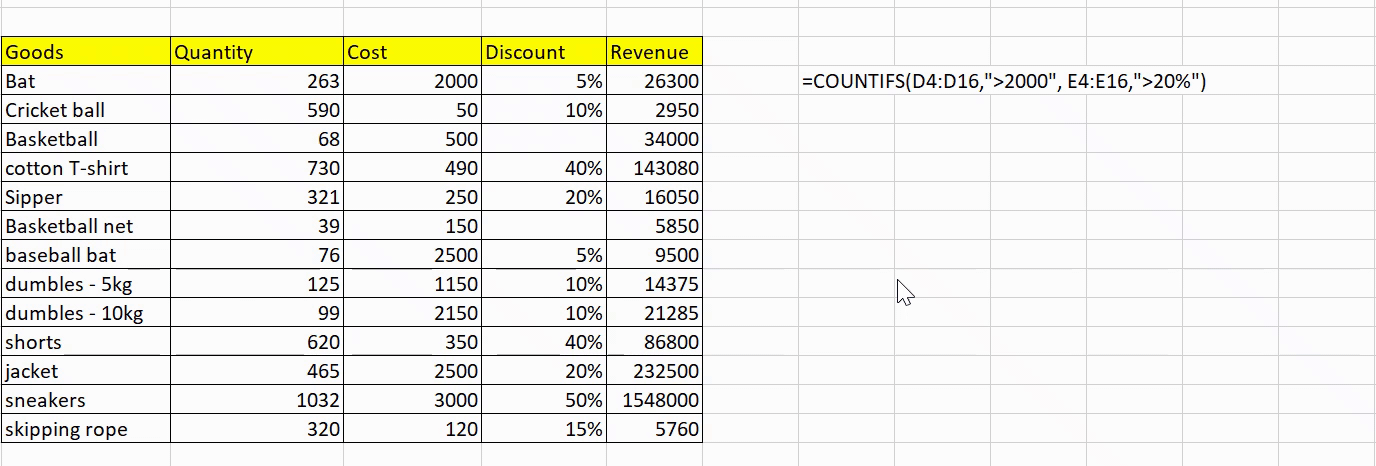The questions seemed complex but it was really easy to find the answer in Excel. Only 1 product i.e., sneakers, cost more than 2000 and sold at a discount rate greater than 20%.
Wonderful, isn’t it? We have gone through some basic statistical functions in MS Excel so far. Next, let’s have a look at the intermediate statistical functions.

## Intermediate Statistical Functions in Excel

We will discuss some of the intermediate statistical functions in MS Excel here related to central tendency and dispersion. These functions are very useful in our day to day life as an analyst.

### 5. Average Function

The most common function we usually use in our daily lives is the average (or mean). The AVERAGE function simply returns the arithmetic mean of all the cells in a given range:

• AVERAGE(number1, [number2], …)
But there’s one simple drawback to using averages – they are prone to outliers. Therefore, they can paint a very unrealistic picture in our analysis. Let’s find out the average number of goods sold:dispersed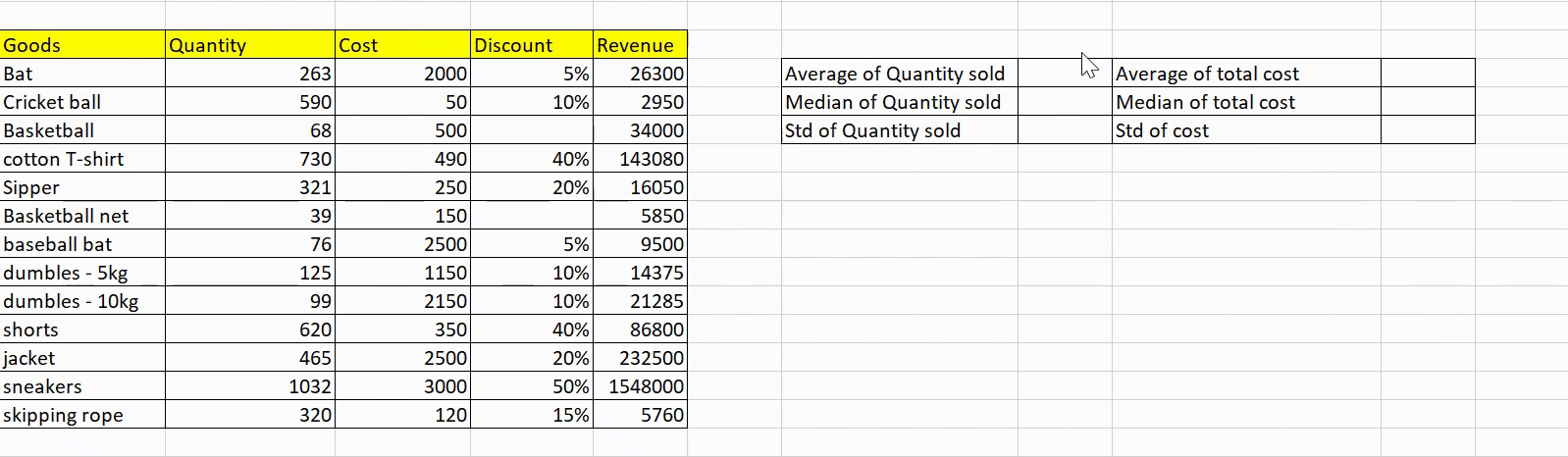The average comes out to be ~ 365.2. We will be doing similar calculations for cost as well.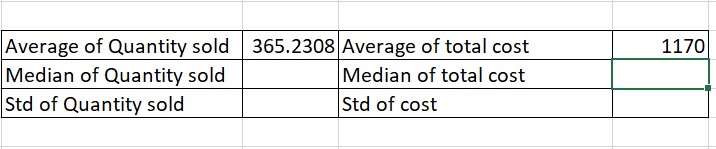### 6. Median Function

The problem of outliers can be solved by using another function for the central tendency – median. The median function returns the middle value of the given range of cells. The syntax is quite simple:

• MEDIAN(number1, [number2], …)
Let’s find the median of the number of goods sold in our sports store and see how close this is to our average value: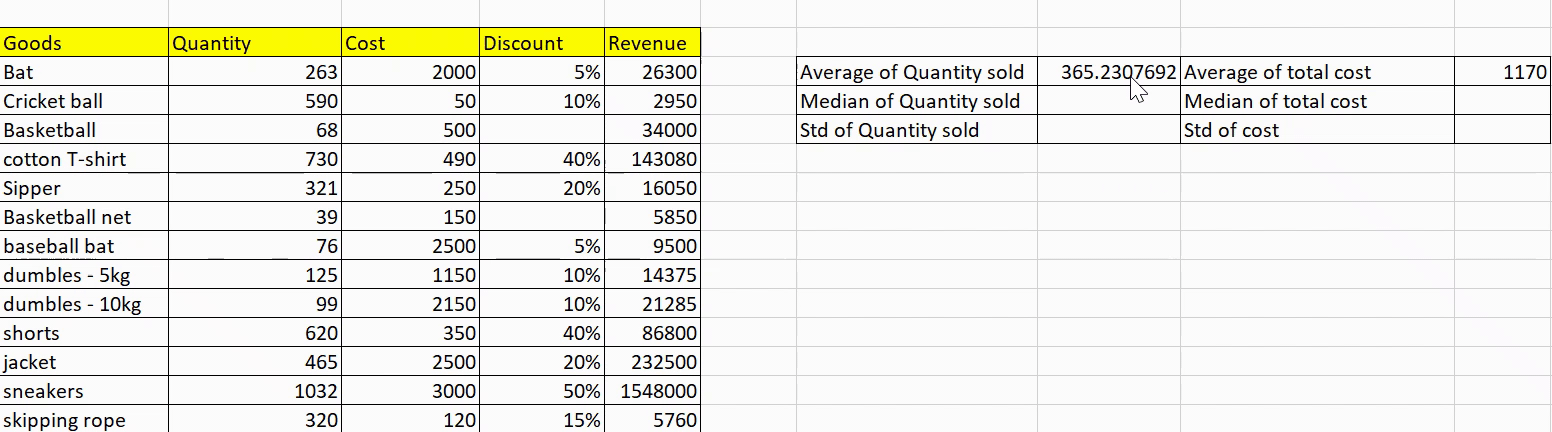We see that the median comes out to be ~ 320 which is pretty close to the average value. It means there is not much fluctuation in our data. Let’s see if this is the case for the cost of goods: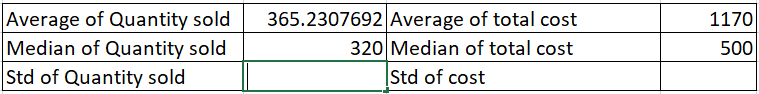The median and the average value for the cost of each item vary a lot. For example, the cost of a ball is 50 but the cost of a bat is 2000 – resulting in high dispersion.

### 7. Mode Function

For numerical values, mean and median usually, suffice but what about categorical values? Here, mode comes into the picture. Mode returns the most frequent and repeated value in the given range of values:

• MODE.SNGL(number1,[number2],…)
Note: MODE.SNGL returns only a single value whereas MODE.MULT returns an array of most commonly occurring values.

Well, this is a simple one. Let’s find the most frequent discount value given by the sports store: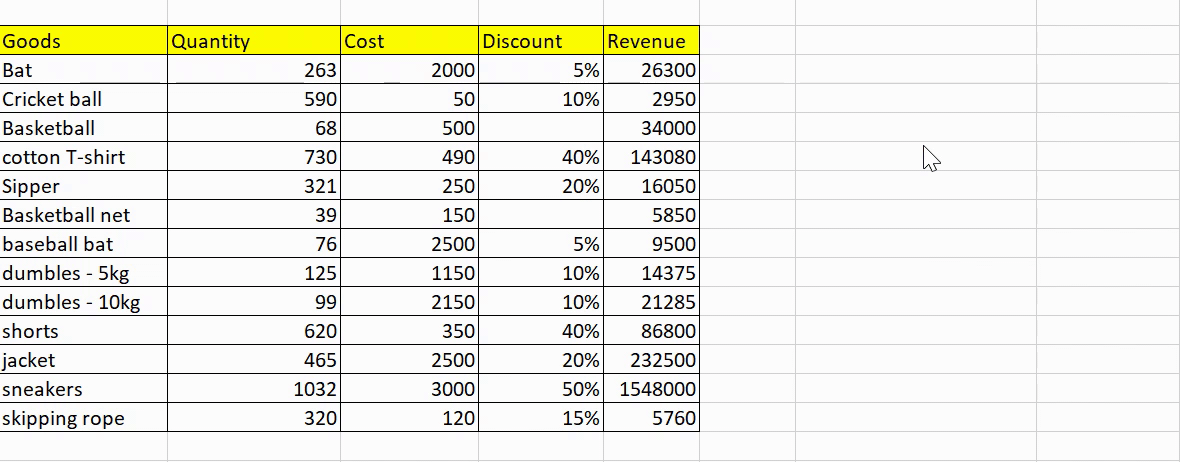This discount value is 10%.

### 8. Standard Deviation Function

Standard Deviation is one of the ways to quantify dispersion. It is a measure of how widely values are dispersed from the average value.

Here, we will be using the STDEV.P function which is used to calculate standard deviation based on the entire population given as arguments:

• STDEV.P(number1,[number2],…)
Note: STDEV.P function assumes that its arguments are the entire population. If that’s not the case, you may use the function STDEV.S() function.
For a large sample size, the standard deviation of the population and samples will return approximately similar values. Previously, we have calculated mean and median to get a picture of the central tendency. Let’s find out the standard deviation to see the level of dispersion: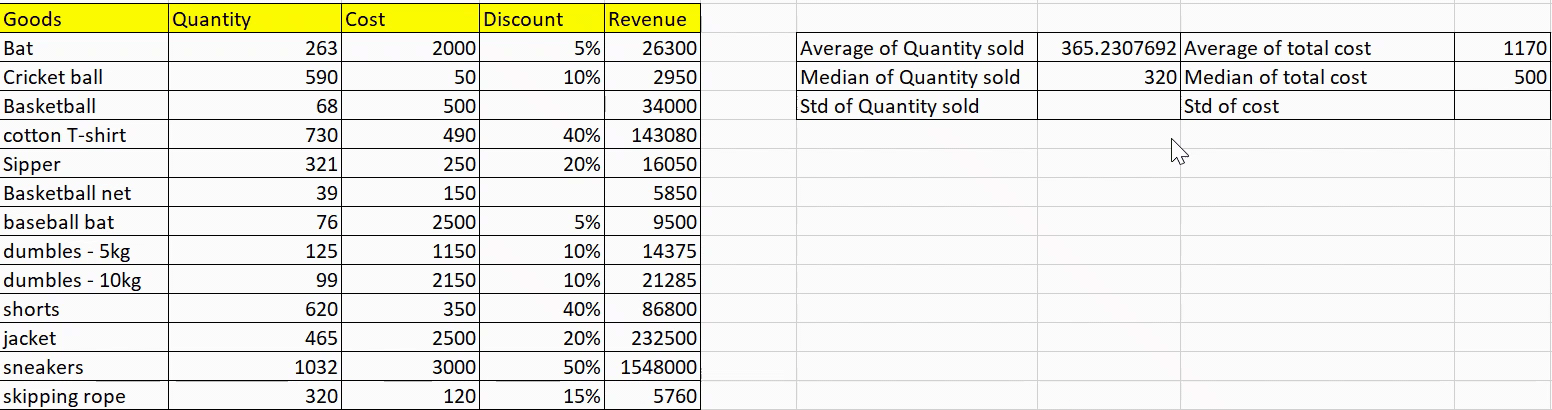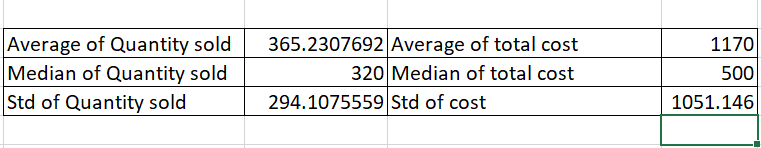As expected, the standard deviation of the quantity sold is less, meaning that the dispersion is less whereas the standard deviation for the cost of products is high.

### 9. Quartiles Functions

This is yet another function with abundant applications in the industry. It helps us divide the population into groups. The QUARTILES.INC returns the quartile of a dataset, based on percentile values from 0 to 1, inclusive.

For example, you can use this function to find out the top 25% of your customer base.

• QUARTILE.INC(array, quart)

### 10. Correlation Function

The CORREL() function is my personal favorite. It provides really powerful insights that are not obvious to the naked eye. The CORREL function returns the correlation coefficient of two cell ranges. But what is that? It basically tells us how strong the relationship is between the two variables.

Note: It does not portray any cause and effect relationship.

• CORREL(array1, array2)

The range of correlation value is between -1 and 1.

Let’s head to our final and most interesting question – is there any relationship between the number of goods sold and the percentage of discount?

###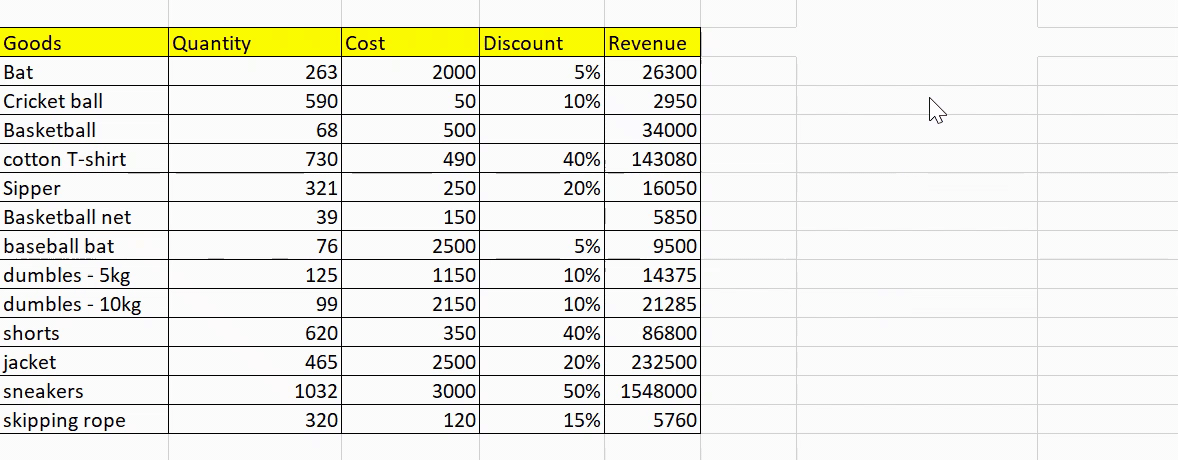Well, the correlation comes out to be ~0.8 which is pretty high. It seems these are positively related – meaning more the discount, more the quantity sold.

## End Notes

We discussed over 10 beginner and intermediate statistical functions in MS Excel in this article ranging from simple count() to advanced correl(). Statistics is one of the most important tools in the kit of an analyst and you can achieve a lot of your statistical goals simply using Excel.

I recommend you go through the following additional resources in Excel:

I will be covering advanced statistical functions in the future. Let me know some of your favorite statistical functions and I will try to incorporate them in my upcoming articles.